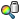# Basic Concepts of Analysis

The software uses the Finite Element Method (FEM). FEM is a numerical technique for analyzing engineering designs. FEM is accepted as the standard analysis method due to its generality and suitability for computer implementation. FEM divides the model into many small pieces of simple shapes called elements effectively replacing a complex problem by many simple problems that need to be solved simultaneously.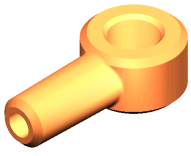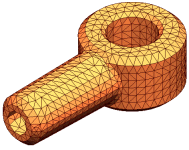CAD model of a part Model subdivided into small pieces (elements)

Elements share common points called nodes. The process of dividing the model into small pieces is called meshing.

The behavior of each element is well-known under all possible support and load scenarios. The finite element method uses elements with different shapes.

The response at any point in an element is interpolated from the response at the element nodes. Each node is fully described by a number of parameters depending on the analysis type and the element used. For example, the temperature of a node fully describes its response in thermal analysis. For structural analyses, the response of a node is described, in general, by three translations and three rotations. These are called degrees of freedom (DOFs). Analysis using FEM is called Finite Element Analysis (FEA).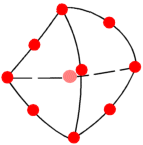A tetrahedral element. Red dots represent nodes. Edges of an element can be curved or straight.

The software formulates the equations governing the behavior of each element taking into consideration its connectivity to other elements. These equations relate the response to known material properties, restraints, and loads.

Next, the program organizes the equations into a large set of simultaneous algebraic equations and solves for the unknowns.

In stress analysis, for example, the solver finds the displacements at each node and then the program calculates strains and finally stresses.

The software offers the following types of studies:

Study type Study icon
Static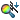Modal Time History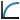Frequency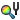Harmonic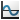Buckling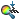Random Vibration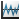Thermal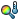Response Spectrum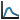Design StudyDrop Test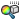Nonlinear Static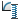Fatigue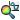Nonlinear DynamicPressure Vessel Design